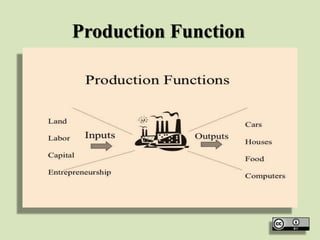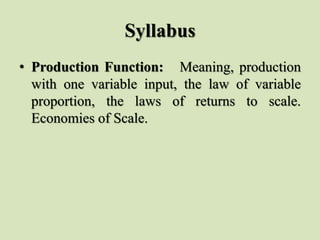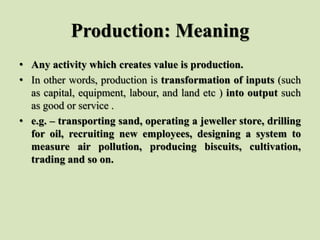Successfully reported this slideshow.

# PRODUCTION FUNCTION PPT.pptx×

# PRODUCTION FUNCTION PPT.pptx

Production Function:   Meaning, production with one variable input, the law of variable proportion, the laws of returns to scale. Economies of Scale.
Production Function:   Meaning, production with one variable input, the law of variable proportion, the laws of returns to scale. Economies of Scale
Production Function:   Meaning, production with one variable input, the law of variable proportion, the laws of returns to scale. Economies of Scale
Production Function:   Meaning, production with one variable input, the law of variable proportion, the laws of returns to scale. Economies of Scale
Production Function:   Meaning, production with one variable input, the law of variable proportion, the laws of returns to scale. Economies of Scale

Production Function:   Meaning, production with one variable input, the law of variable proportion, the laws of returns to scale. Economies of Scale

Production Function:   Meaning, production with one variable input, the law of variable proportion, the laws of returns to scale. Economies of Scale

Production Function:   Meaning, production with one variable input, the law of variable proportion, the laws of returns to scale. Economies of Scale

Production Function:   Meaning, production with one variable input, the law of variable proportion, the laws of returns to scale. Economies of Scale.
Production Function:   Meaning, production with one variable input, the law of variable proportion, the laws of returns to scale. Economies of Scale
Production Function:   Meaning, production with one variable input, the law of variable proportion, the laws of returns to scale. Economies of Scale
Production Function:   Meaning, production with one variable input, the law of variable proportion, the laws of returns to scale. Economies of Scale
Production Function:   Meaning, production with one variable input, the law of variable proportion, the laws of returns to scale. Economies of Scale

Production Function:   Meaning, production with one variable input, the law of variable proportion, the laws of returns to scale. Economies of Scale

Production Function:   Meaning, production with one variable input, the law of variable proportion, the laws of returns to scale. Economies of Scale

Production Function:   Meaning, production with one variable input, the law of variable proportion, the laws of returns to scale. Economies of Scale

### PRODUCTION FUNCTION PPT.pptx

1. 1. Production Function
2. 2. Syllabus • Production Function: Meaning, production with one variable input, the law of variable proportion, the laws of returns to scale. Economies of Scale.
3. 3. Production: Meaning • Any activity which creates value is production. • In other words, production is transformation of inputs (such as capital, equipment, labour, and land etc ) into output such as good or service . • e.g. – transporting sand, operating a jeweller store, drilling for oil, recruiting new employees, designing a system to measure air pollution, producing biscuits, cultivation, trading and so on.
4. 4. Production-Function • In economics, the technical law, relating inputs to outputs, has been given the name of production- function. • In simple words, production - function expresses the relationship between the physical inputs and physical output of a firm for a given state of technology. • The production-function can be written mathematically as follows: • qx = f (F1 , F2 , F3 ……….. Fn) • Here, qx = the quantity of x commodity • F1 , F2 , F3 ……….. Fn = Different factor-inputs • This equation tells that the output of x depends on the factors F1 , F2 , F3 ……….. Fn ,etc. • There is functional relationship between factor-inputs and the amount of goods x. • For example, the output of cloth depends on cotton, thread, machine, labour, chemicals, etc. • Hence the relationship between factor-inputs (e.g. thread, machine, labour, chemicals, etc.) and the output of cloth can be shown with the help of production- function.
5. 5. Production Function • Production function express the technological relationship between physical inputs and physical output of a firm under given technology. • A production function may be write as follows Where, • Q = output (total product) • Q = f(N, L, K, E,…..) • N (land), L(labour), K(capital), E(entrepreneurship) , .. are the inputs.
6. 6. Production Function
7. 7. Inputs (Factors) of Production/ Factor Inputs/Factors/Inputs • Factors of production are broadly classified as : • Land: • Anything which is gift of nature and not the result of human effort, e.g. soil, water, forests, minerals. Owner of land is called landlord. Reward of land is called as rent. • Labour: • Physical or mental effort of human beings that undertakes the production process. Labour is supplied by the workers. Labour can be skilled as well as unskilled, physical or intellectual. • Reward/price of labour is called as wages/ salary.
8. 8. Factors Of Production
9. 9. Inputs (Factors) of Production/ Factor Inputs/Factors/Inputs • Capital: • Wealth which is used for further production as machine/ equipment/intermediary good. It is outcome of human efforts meaning capital is man-made. Reward of capital is called as interest, • Enterprise/Entrepreneurship/organisation: • The ability and action to take risk of collecting, coordinating, and utilizing all the factors of production for the purpose of uncertain economic gains. Owner of enterprise is entrepreneur. Reward of entrepreneurship is called as profit.
10. 10. Concept of Time • Alfred Marshal introduced the element of time in production decision. Time can categorize as under: • Market Period or Very Short Period: • Market Period or Very Short Period is a period during which all factors of production and hence cost remains fixed. As such, outputs as well as supply also remain fixed. • Short Run: • Short run a period so brief that the amount of at least one input is fixed. Thus we have both fixed as well as variable factors. • Long Run: • Long Run is a period of time sufficient enough for all inputs (or factors of production), to be variable as far as an individual firm is concerned. • The length of time necessary for all inputs to be variable may differ according to the nature of the industry and the structure of a firm.
11. 11. Concept of Time Marshall defined 4 time periods: Market period - Very short period in which supply is fixed (perfectly inelastic). No reflex action of price on quantity supplied. Short run - A period in which the firm can change production and supply but cannot change plant size. Higher prices cause larger quantities to be supplied.
12. 12. Concept of Time Long run - Plant size can vary and all costs become variable Secular period - (Very long run) Permits technology and population to vary
13. 13. Types of Production-Function Before analyzing the types of production-function it will be useful to understand the meaning of following important terms : A. Fixed Factors and Variable Factors • Factors of production are broadly classified into two categories i.e. fixed and variable factors: • (i) Fixed Factors - The factor inputs which cannot be varied in the short-period, as and when required are called fixed factors. • Examples of Fixed Factors are : Plant, machinery, heavy equipments, factory building, land etc. • (ii) Variable Factors - The factor inputs which can easily be varied, in the short-period as and when required, are called variable factors. • Examples of variable factors are : labour, raw material, power, fuel etc. • The distinction between fixed factors and variable factors appears only in the short-period. In the long-run, all the factors of production become variable factors.
14. 14. Fixed Factors and Variable Factors
15. 15. Types of Production-Function • Short Period And Long Period • The time-period during which a firm in order to make changes in its production can change only in its variable factors but not in its fixed factors, is termed as short-period. In the short-period, a firm cannot change its scale of plant. • The time period in which a firm can change all the factors of production and its scale of plant, is termed as long-period. • In economics, we study two types of production- functions. In other words, there are two kinds of input-output relations in production-functions.
16. 16. Types of Production-Function
17. 17. Types of Production-Function
18. 18. Types of Production-Function (i) Short-run Production-functions or the Law of Variable Proportions • In the short period, some factors are fixed and some of them are variable. What happens when additional units of one variable factor of production are combined with a fixed stock of some factors of production, is discussed under short-run production- functions. The law which tells about this relation is called the law of variable proportions or returns to a factor. Since it is related to a short-period, it is called short-run production-function.
19. 19. Law of Variable Proportions
20. 20. Law of Variable Proportions
21. 21. Types of Production-Function ii) Long-run Production-function or Returns to Scale • In the long run, all factor-inputs can be varied. It means, that in the long-run, we can expand or reduce the scale of production as well. The way in which the output varies with the changes in the scale of production is discussed in the long-run production-functions. The law which states this relationship is also called returns to scale. Since it is related to the long-period, it is called long-run production-function.
22. 22. Returns to Scale
23. 23. Returns to Scale
24. 24. Types of Production-Function • In this context we have to define three key terms :- • (1) Total Product - It refers to the total output of the firm per period of time • (2) Average Product - Average Product is total output per unit of the variable input. Thus Average Product is total product divided by the number of units of the variable factor. • AP = Q/L where Q is Total Product, L is the quantity of labour. • (3) Marginal Product - Marginal Product is the change in total product resulting from using • an additional unit of the variable factor. • MP = dQ/dL, where d is the rate of change
25. 25. Concept of Product • There are three concepts of product such as: • Total Product • Average Product • Marginal Product
26. 26. Total Product • Total Product refers (TP) to the total volume of goods and services produced by a firm during a given period of time. • Where L is quantity of a factor • AP is the average productivity of a factor. • MP is marginal productivity of a factor .
27. 27. Average Product • Average Product (AP) is output (total product) per unit of a factor. Where, • i.e. AP • Q is total product • L is quantity of a factor input
28. 28. Marginal Product • Marginal Product (MP) is rate of change in total product with respect to a factor. • Concept of Product contd. • In other words, marginal product is the addition to total product by utilizing one more one unit of variable input to the production process, keeping other factor fixed. Where , • dQ is change in total product • dL is change in quantity of a factor input.
29. 29. Law of Variable Proportions or Returns to a Factor Meaning and Definition • The law of variable proportions has an important place in economic theory. • This law exhibits the short-run production-functions in which one factor is variable and others are fixed. • The extra output obtained by applying extra unit of a variable factor can be greater than, equal to or less than the output obtained by its previous unit. • If the number of units of a variable factor is increased, the way wherein the output changes is the concern of this law.
30. 30. Law of Variable Proportions or Returns to a Factor • Thus it refers to the effect of changing factor-ratio on the output. • In short, the law which exhibits the relationship between the units of a variable factor (keeping all other factors as constant) and the amount of output in the short-run is known as returns to a variable factor. • Thus the law of variable proportions is also named as (or returns to a factor) returns to a variable factor. • The law states that with the increase in a variable factor, keeping other factors constant, total product increases at an increasing rate, then increases at diminishing rate and finally starts declining.
31. 31. Reason as to why it is called the Law of Variable Proportions: • The factor- proportion (or factor-ratio) varies as one input varies and all others are constant. • This can be understood with the help of an example. • Suppose in the beginning 10 acres of land and 1 unit of labour are taken for production, hence land-labour are taken for production, hence land-labour ratio was 10: 1. • Now if the land remains the same but the units of labour increases to 2, now the land-labour ratio would become 5: 1.
32. 32. Reason as to why it is called the Law of Variable Proportions: • Thus, this law analyses the effects of change in factor-proportions on the amount of output and is, therefore, called the law of variable proportions.
33. 33. Explanation of the Law • The law of variable proportions can be illustrated with the help of the following example and diagram
34. 34. Explanation of the Law • In this example, we assume that land is the fixed factor and labour is a variable factor. • The table shows the different amounts of output obtained by applying different units of labour to one acre of land which continues to be fixed.
35. 35. Diagram • The law of variable proportions can be explained with the help of diagram below. • In order to make simple presentation we have drawn a TPP (Total Physical Product) curve and a MPP (Marginal Physical Product) curve as smooth curves in the diagram, against the variable input, labour.
36. 36. Three Stages of the Law
37. 37. Three Stages of the Law
38. 38. Significance of the Three Stages of the Law • With the knowledge of the three stages of the law, a producer can choose the appropriate stage of its operation
39. 39. Reason for Operation of the Law • In the short-period all factors of production cannot be varied. • Here one is variable factor and others are fixed factors. • There is an optimum combination of different factors that gives the maximum output. • When there is increase in the units of a variable factor before the point of optimum combination, the factor proportion becomes more suitable and fixed factors are more efficiently utilized. • Hence it increases the marginal physical product. • In the initial stages the total product may rise at an increasing rate when we employ more units of a variable factor to the fixed factors.
40. 40. Reason for Operation of the Law • But later, when we employ more units of a variable factor beyond this optimum combination, the factor proportion becomes unsuitable and inefficient; hence the marginal product of that variable factor declines. • The quantity of the fixed factor-input per unit of the variable input falls as more and more of the latter is put to use. • Successive units of the variable input, therefore, must add decreasing amounts to the total output as they have less of the fixed input to work with.
41. 41. Returns to Scale • In the long run, all factors are variable. • The expansion of output may be achieved by varying all factor-inputs. • When there are changes in all factor-inputs in the same proportion, the scale of production (or the scale of operation) also gets changed. • Thus, the change in scale means that all factor inputs are changed in the same proportion. • The term returns to scale refers to the changes in output as all factor-inputs change in the same proportion in the long run. • The law expressing the relationship between varying scales of production (i.e. change of all factor inputs in the same proportion) and quantities of output. • The increase in output may be more than, equal to, or less than proportional to the increase in factor-inputs. • Accordingly, returns to scale are also of three types - increasing returns to scale, constant returns to scale and diminishing returns to scale.
42. 42. Returns to Scale • The law of returns to scale with its all the three stages (or types) is shown in the following example and diagram below:
43. 43. Returns to Scale
44. 44. Returns to Scale
45. 45. Returns to Scale • The law of returns to scale can also be shown with the help of a very simple diagram which is given below. From A to B in the diagram is the stage of increasing returns; from B to C constant returns, and from C to D is the diminishing returns to scale.
46. 46. Causes for the Operation of Returns to Scale • Returns to scale occur mainly because of two reasons
47. 47. Causes for the Operation of Returns to Scale
48. 48. Causes for the Operation of Returns to Scale • Thus, the main reason for the operation of the different forms of returns to scale is found in economies and diseconomies. • — When economies exceed the diseconomies → the stage of increasing returns operate. • — When economies equal diseconomies → the stage of constant returns to scale. • — when diseconomies exceed the economies → stage of diminishing returns to scale.
49. 49. Distinction between Returns to a Variable Factor (or Law of Variable Proportions) and Returns to Scale • The main differences between returns to a variable factor and returns to scale are as indicated below:
50. 50. Thanks…